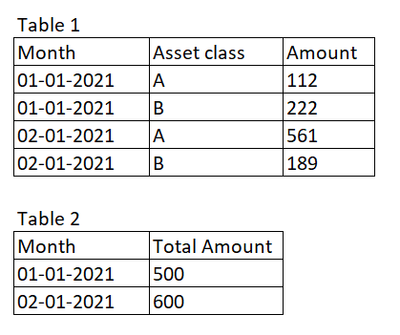# New to Qlik Sense

If you’re new to Qlik Sense, start with this Discussion Board and get up-to-speed quickly.

Announcements
Qlik Cloud Maintenance is scheduled between March 27-30. Visit Qlik Cloud Status page for more details.
cancel
Showing results for
Did you mean:Contributor III

## How to create a stacked bar chart coming from 2 tables - Qlik sense

Hi All!

I have a two tables. I have created stacked bar chart from 1 table. I want to add one more on this stacked bar chart and the amount is coming from a different table.Stacked bar chart from table 1:Desired Output:Thank you!

1 Solution

Accepted SolutionsPartner - Master III

Hi ,

the bar chart has this limitation :
you can either use 2 dimensions and 1 measure or 1 dimension and as many measures you want.
you have 2 options:
1 concatenate the two tables in the load script :

``````Table1:
[Asset class],
Amount
From XXX;
concatenate (Table1)
'Others' as [Asset class]
[Total Amount] as Amount
From YYY;``````

Then you'll have a simple chart :
1st dimension: Month
2nd dimension:  Asset class
measure: Sum(Amount)
your second option is to leave the table as they are and to build this chart.
dimension: Month

1st measure: sum(if([Asset class]='A', Amount)

2nd measure: sum(if([Asset class]='B', Amount)

3rd measure: sum([Total Amount])

2 RepliesPartner - Master III

Hi ,

the bar chart has this limitation :
you can either use 2 dimensions and 1 measure or 1 dimension and as many measures you want.
you have 2 options:
1 concatenate the two tables in the load script :

``````Table1:
[Asset class],
Amount
From XXX;
concatenate (Table1)
'Others' as [Asset class]
[Total Amount] as Amount
From YYY;``````

Then you'll have a simple chart :
1st dimension: Month
2nd dimension:  Asset class
measure: Sum(Amount)
your second option is to leave the table as they are and to build this chart.
dimension: Month

1st measure: sum(if([Asset class]='A', Amount)

2nd measure: sum(if([Asset class]='B', Amount)

3rd measure: sum([Total Amount])Contributor III
Author

Hi @lironbaram thank you for the solution!! I used combo chart and added 3 measures with 3 different colours.Tags
Community Browser